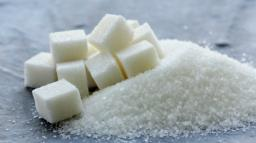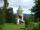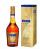# Sugar cubes

The glass has 600 ml of tea, which represents 80% of the volume of the glass. If you put twenty regular sugar cubes of 2 cm in the tea, how many ml of tea are poured?

Correct result:

x =  10 ml

#### Solution:We would be pleased if you find an error in the word problem, spelling mistakes, or inaccuracies and send it to us. Thank you!Tips to related online calculators
Do you know the volume and unit volume, and want to convert volume units?

## Next similar math problems:

• Here isHere is a data set (n=117) that has been sorted. 10.4 12.2 14.3 15.3 17.1 17.8 18 18.6 19.1 19.9 19.9 20.3 20.6 20.7 20.7 21.2 21.3 22 22.1 22.3 22.8 23 23 23.1 23.5 24.1 24.1 24.4 24.5 24.8 24.9 25.4 25.4 25.5 25.7 25.9 26 26.1 26.2 26.7 26.8 27.5 27.6 2
• VintnerHow high can vintner fill keg with crushed red grapes if these grapes occupy a volume of 20 percent? Keg is cylindrical with a diameter of the base 1 m and a volume 9.42 hl. Start from the premise that says that fermentation will fill the keg (the number
• The tentThe tent shape of a regular quadrilateral pyramid has a base edge length a = 2 m and a height v = 1.8 m. How many m2 of cloth we need to make the tent if we have to add 7% of the seams? How many m3 of air will be in the tent?
• Fuel economyHow many kilometers is sufficient petrol in the cylinder fuel tank with a diameter 40 cm and the base of tank length 1 m, when it is filled to 60% and if the car consume 15 liters per 100 km?
• The coilHow many ropes (the diameter 8 mm) fit on the coil (threads are wrapped close together) The coil has dimension: the inner diameter 400mm, the outside diameter 800mm and the length of the coil is 470mm
• Pyramid cutWe cut the regular square pyramid with a parallel plane to the two parts (see figure). The volume of the smaller pyramid is 20% of the volume of the original one. The bottom of the base of the smaller pyramid has a content of 10 cm2. Find the area of the
• Cube into sphereThe cube has brushed a sphere as large as possible. Determine how much percent was the waste.
• The ballThe ball has a radius of 2m. What percentage of the surface and volume is another sphere whose radius is 20% larger?
• Church roof 2The roof has the shape of a rotating cone shell with a base diameter of 6 m and a height of 2.5 m. How many monez (CZK) will cost the roof cover sheet if 1 m2 of metal sheet costs 152 CZK and if you need 15% extra for joints, overlays and waste?
• Four prismsQuestion No. 1: The prism has the dimensions a = 2.5 cm, b = 100 mm, c = 12 cm. What is its volume? a) 3000 cm2 b) 300 cm2 c) 3000 cm3 d) 300 cm3 Question No.2: The base of the prism is a rhombus with a side length of 30 cm and a height of 27 cm. The heig
• Cuboid enlargementBy how many percent increases the volume of cuboid if its every dimension increases by 30%?
• TanksFire tank has cuboid shape with a rectangular floor measuring 13.7 m × 9.8 m. Water depth is 2.4 m. Water was pumped from the tank into barrels with a capacity of 2.7 hl. How many barrels were used, if the water level in the tank fallen 5 cm? Write
• CylinderIn a 1-meter diameter cylinder is 1413 liters of water, which is 60% of the cylinder. Calculate the cylinder height in meters, do not write the units. The resulting value round and write as an integer.
• HectolitersHow deep is the pool if there are 2025 hectoliters of water and the bottom dimensions are a = 15 meters b = 7,5 meters and the water level is up to 9/10 (nine-tenths) of height.
• Roof 8How many liters of air are under the roof of tower which has the shape of a regular six-sided pyramid with a 3,6-meter-long bottom edge and a 2,5-meter height? Calculate the supporting columns occupy about 7% of the volume under the roof.
• AlcoholHow many cubic centimeters of 96% alcohol is needed to obtain 0.75 liter of 40% alcohol?
• PeroxideHow many ml 30% of peroxide (H2O2) should be poured into 100ml H2O to give a 20% solution?